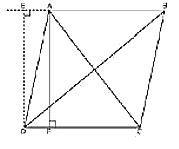Guru

# Prove that the sum of the squares of the diagonals of parallelogram is equal to the sum of the squares of its sides. Q.6

• 0

The best question of optional chapter of class 10th math of chapter name Triangles of exercise 6.6, Give me the best way to find this question Prove that the sum of the squares of the diagonals of parallelogram is equal to the sum of the squares of its sides

Share

1. Let us consider, ABCD be a parallelogram. Now, draw perpendicular DE on extended side of AB, and draw a perpendicular AF meeting DC at point F.By applying Pythagoras Theorem in ∆DEA, we get,

DE2 + EA2 = DA2 ……………….… (i)

By applying Pythagoras Theorem in ∆DEB, we get,

DE2 + EB2 = DB2

DE2 + (EA + AB) 2 = DB2

(DE2 + EA2) + AB2 + 2EA × AB = DB2

DA2 + AB2 + 2EA × AB = DB2 ……………. (ii)

By applying Pythagoras Theorem in ∆ADF, we get,

AD2 = AF2 + FD2

Again, applying Pythagoras theorem in ∆AFC, we get,

AC2 = AF2 + FC2 = AF2 + (DC − FD) 2

= AF2 + DC2 + FD2 − 2DC × FD

= (AF2 + FD2) + DC2 − 2DC × FD AC2

AC2= AD2 + DC2 − 2DC × FD ………………… (iii)

Since ABCD is a parallelogram,

AB = CD ………………….…(iv)

And BC = AD ………………. (v)

In ∆DEA and ∆ADF,

∠DEA = ∠AFD (Each 90°)

∴ ∆EAD ≅ ∆FDA (AAS congruence criterion)

⇒ EA = DF ……………… (vi)

Adding equations (i) and (iii), we get,

DA2 + AB2 + 2EA × AB + AD2 + DC2 − 2DC × FD = DB2 + AC2

DA2 + AB2 + AD2 + DC2 + 2EA × AB − 2DC × FD = DB2 + AC2

From equation (iv) and (vi),

BC2 + AB2 + AD2 + DC2 + 2EA × AB − 2AB × EA = DB2 + AC2

AB2 + BC2 + CD2 + DA2 = AC2 + BD2

• 0